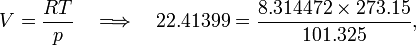# Mole (unit)

In chemistry and physics, the mole is an SI base unit of amount of substance, used to signify how much or how many--just as one would use "one kilogram" or "one dozen". The unit is abbreviated mol. The word "mole" is derived from "gram molecular weight", the original term. Industrial chemists and chemical engineers also use a "kilogram molecular weight" as equal to 1 kmol (or kg-mol) and in the United States as a pound-mol (lb-mol) which is equal to 453.592 gram mols.

Informally the mole may be defined for a pure (i.e., consisting of one kind of molecules) substance by the easily remembered sentence:

the mole is the amount of substance that weighs (in grams) as much as its molecular weight. 

For instance, the molecular weight of the water molecule (H2O) is 18.02, and therefore one mole of pure water weighs 18.02 gram.

The definition of a mole extends to different entities: molecules, ions, atoms, electrons - any elementary substance in chemistry. The formal SI definition reads:

the mole is the amount of substance of a system which contains as many elementary entities as there are atoms in 0.012 kilogram of 12C (carbon-12).

The definition of the mole leads to the definition of Avogadro's constant: NA is the number of entities (e.g. molecules or atoms) in one mole. NA ≈ 6×1023/mol.

One mole of an ideal gas occupies 22.41399 litres at an absolute temperature T of 273.15 K = 0 °C and pressure p of 101.325 kPa = 1 atm. This follows from the ideal gas equation:$V = \frac{RT}{p} \quad \Longrightarrow\quad 22.41399 = \frac{8.314472\times 273.15}{101.325},$

where R ( = 8.314472 J mol−1 T −1 ) is the molar gas constant. A mole of a real gas will deviate from this volume, but for many real gases 22.4 litres for a mole is a good—first—approximation.

### Examples

The total mass of an amount of substance is the sum of the masses of its entities. For example, consider a pure substance B of entities with molecular mass M(B) u ( u is unified atomic mass unit). Recalling that

1 u = 1/NA gram,

we find that one mole of B weighs NA × M(B) u = M(B) gram. For example, NA molecules of water (B = H2O) have mass 18.02 gram.

Let us give another example. The standard atomic weight of magnesium M(Mg) = 24.3050 u, which is the mass of a magnesium atom averaged over its isotopes weighted by natural abundance. One mole of magnesium is NA × M(Mg) u = 24.3050 gram. This example shows that the atomic mass of any element can be interpreted in two ways: (1) the average mass of a single atom in unified atomic mass units (u) or (2) the average mass of a mole of atoms in grams. For magnesium, (1) the average mass of a single magnesium atom is 24.3050 u or (2) the average mass of a mole of magnesium atoms is 24.3050 gram.

Further examples: a mole of hydrogen molecule, standard atomic weight of H is 1.00794, has the mass 2×1.00794 = 2.01588 gram. A mole of oxygen, standard atomic weight 15.9994, has the mass 31.9988 gram. 

To explain the usefulness of the mole concept we consider the following example of a chemical reaction:

2 A + 6 B → 2 AB3

This equation is expressed in numbers of atoms and molecules, which are impractical to measure or count directly. However, if we multiply the equation by Avogodro's number, it is expressed in macroscopic quantities. The equation tells us then that 2 moles of A react with 6 moles of B to give 2 moles of AB3.

In general, the molecular masses of the compounds A [ = M(A)] and B [ = M(B)] are known and hence also is the molecular mass of AB3 [ = M(AB3)]. The reaction equation can be translated thus: 2M(A) gram of A reacts with 6M(B) gram of B to give 2M(AB3) gram of AB3.

A real life example:

2H2 + O2 → 2H2O

Using rounded numbers: 4 gram H2 reacts with 32 gram O2 giving 36 gram H2O.